# On the average order of the gcd-sum function over arbitrary sets of integers

V. Siva Rama Prasad and P. Anantha Reddy
Notes on Number Theory and Discrete Mathematics
Print ISSN 1310–5132, Online ISSN 2367–8275
Volume 27, 2021, Number 3, Pages 16-28
DOI: 10.7546/nntdm.2021.27.3.16-28

## Details

### Authors and affiliations

V. Siva Rama PrasadProfessor (Retired), Department of Mathematics, Osmania University

P. Anantha ReddyGovernment Polytechnic

### Abstract

Let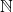denote the set of all positive integers and for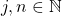, let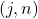denote their greatest common divisor. For any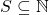, we define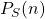to be the sum of those, where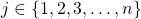. An asymptotic formula for the summatory function ofis obtained in this paper which is applicable to a variety of sets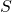. Also the formula given by Bordellès for the summatory function of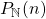can be derived from our result. Further, depending on the structure of, the asymptotic formulae obtained from our theorem give better error terms than those deducible from a theorem of Bordellès (see Remark 4.4).

### Keywords

• Pillai function
• gcd-sum function
• Asymptotic formula
• Möbius function of S
• Dirichlet product
• r-free integer
• Semi-r-free integer
• (k, r)-integer
• Unitary divisor

### 2020 Mathematics Subject Classification

• Primary: 11A25
• Secondary: 11N37

### References

1. Apostol, T. M. (1998). Introduction to Analytic Number Theory, Springer International Student Edition, Narosa Publishing House, New Delhi.
2. Bordellès, O. (2007). A note on the average order of the gcd-sum function. Journal of Integer Sequences, 10, Article 07.3.3.
3. Bordellès, O. (2010). The composition of the gcd and certain arithmetic functions. Journal of Integer Sequences, 13, Article 10.7.1.
4. Bourgain, J., & Watt, N. (2017). Mean Square of zeta function, circle problem and divisor problem revisited. Preprint. Available online at: arXiv:1709.04340v1 [math.AP].
5. Broughan, K. A. (2001). The gcd-sum function. Journal of Integer Sequences, 4, Article 01.2.2.
6. Cohen, E. (1959). Arithmetical functions associated with arbitrary sets of integers. Acta Arithmetica, 5, 407–415.
7. Cohen, E. (1961). Some sets of integers related to the k-free integers. Acta Scientiarum Mathematicarum (Szeged), 22, 223–233.
8. Hardy, G. H. (1916). The average order of the arithmetical functions P(x) and (x). Proceedings of the London Mathematical Society, 15(2), 192–213.
9. Pillai, S. S. (1933). On an arithmetic function. Journal of the Annamalai University, 2, 243–248.
10. Rieger, G. J. (1973). Einige Verteilungsfragen mit k-leeran Zahlen, r-Zahlen und Primzahlen. Journal für die reine und angewandte Mathematik, 262/263, 189–193.
11. Subbarao, M. V., & Suryanarayana, D. (1974). On the order of the error function of the (k, r)-integers. Journal of Number Theory, 6(2), 112–123.
12. Suryanarayana, D., & Sitaramachandra Rao, R. (1973). Distribution of semi-k-free integers, Proceedings of the American Mathematical Society, 37(2), 340–346.
13. Suryanarayana, D., & Siva Rama Prasad, V. (1971). The number of k-free divisors of an integer. Acta Arithmetica, XVII, 345–354.
14. Tóth, L. (2010). A survey of gcd-sum functions. Journal of Integer Sequences, 13, Article 10.8.1.
15. Tóth, L. (2011). Weighted gcd-sum function. Journal of Integer Sequences, 14, Article 11.7.7.
16. Walfisz, A. (1963). Weylsche Exponentialsummen in der neueren Zahlentheorie. Leipzig B. G. Teubner.

## Cite this paper

Siva Rama Prasad, V., & Anantha Reddy, P. (2021). On the average order of the gcd-sum function over arbitrary sets of integers. Notes on Number Theory and Discrete Mathematics, 27(3), 16-28, doi: 10.7546/nntdm.2021.27.3.16-28.